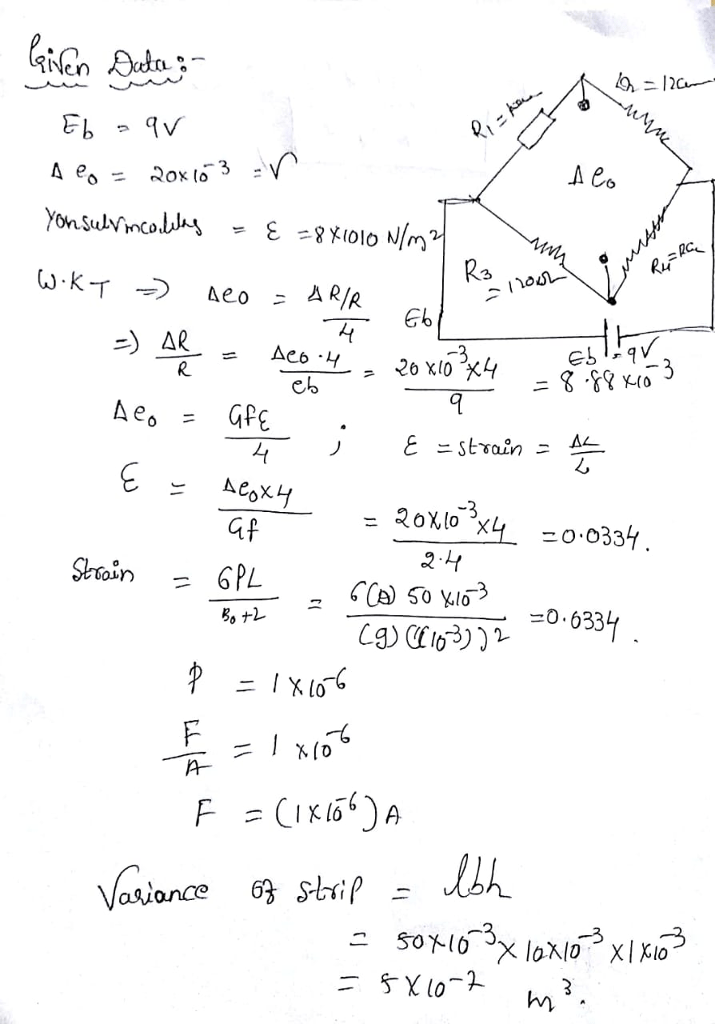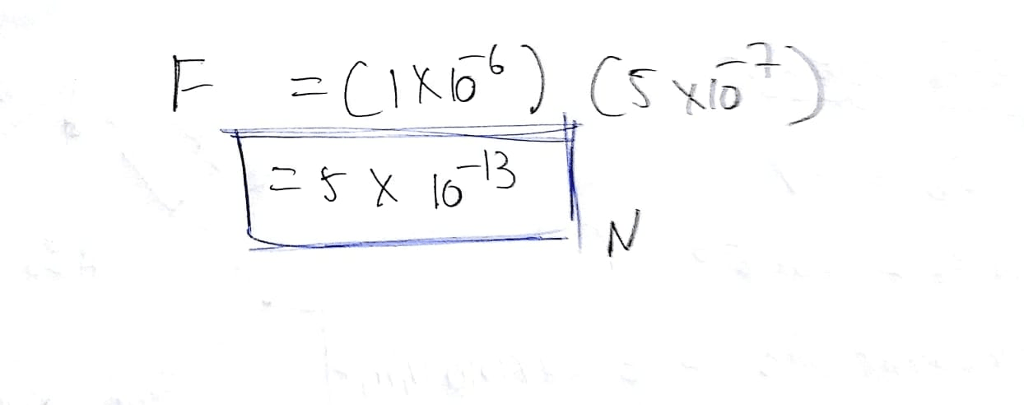# In the resistance strain gauge arrangement of Figure 4.72 strain gauge resistance R1=R2=R3=R4=120 ohm Eb=9V.If the output voltage of the bridge is 20mV,find the value of force applied to the strip.Thickness t of the strip=1 mm.Width b of strip=10mm.Young's modulus E of strip material=8 x 10^10 N/m^2.Length L=50 mm.Strain at root of cantilever=6 PL/Ebt^2.Assume output resistance as infinity.Gauge factor=2.4.

Question-AnswerCategory: Measurement & InsturmentationIn the resistance strain gauge arrangement of Figure 4.72 strain gauge resistance R1=R2=R3=R4=120 ohm Eb=9V.If the output voltage of the bridge is 20mV,find the value of force applied to the strip.Thickness t of the strip=1 mm.Width b of strip=10mm.Young's modulus E of strip material=8 x 10^10 N/m^2.Length L=50 mm.Strain at root of cantilever=6 PL/Ebt^2.Assume output resistance as infinity.Gauge factor=2.4.

In the resistance strain gauge arrangement of Figure 4.72 strain gauge resistance R1=R2=R3=R4=120 ohm Eb=9V.If the output voltage of the bridge is 20mV,find the value of force applied to the strip.Thickness t of the strip=1 mm.Width b of strip=10mm.Young’s modulus E of strip material=8 x 10^10 N/m^2.Length L=50 mm.Strain at root of cantilever=6 PL/Ebt^2.Assume output resistance as infinity.Gauge factor=2.4.